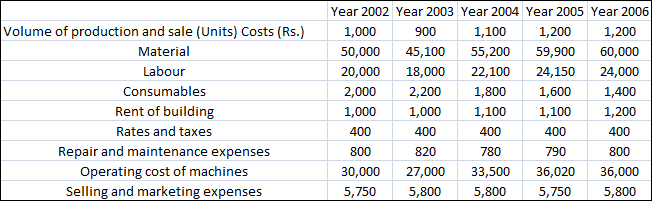### CAT 2007 Question Paper Question 36

Instructions

Directions for the following four questions:

Answer the following questions based on the information given below.

The following table shows the break-up of actual costs incurred by a company in last five years (year 2002 to year 2006) to produce a particular product.The production capacity of the company is 2000 units. The selling price for the year 2006 was Rs. 125 per unit. Some costs change almost in direct proportion to the change in volume of production, while others do not follow any obvious pattern of change with respect to the volume of production and hence are considered fixed. Using the information provided for the year 2006 as the basis for projecting the figures for the year 2007, answer the following questions:

Question 36

# If the company reduces the price by 5%, it can produce and sell as many units as it desires. How many units the company should produce to maximize its profit?

Solution

Fixed costs in 2006 are consumables, rent of building, rates and taxes, repair and maintenance expenses and selling and marketing expenses. We can see this from the trend followed in the years 2002-2006.

Fixed costs in 2006 = 1400 + 1200 + 400 + 800 + 5800 = 9600 => fixed costs in 2007 are same.

We can see that that material , labour and operation costs depend on no. of units.

If we take units = x .

Total cost of these 3 materials is 50x +20x+30x = 100x and remaining fixed cost taking same as in 2006 we get , 9600+100x.

Selling price is reduced by 5% => SP = 125 * 0.95 = Rs 118.75

Profit = 118.75x - 100x - 9600 = 18.75x - 9600

Profit will be maximum when x is maximum

=> 2000 units.